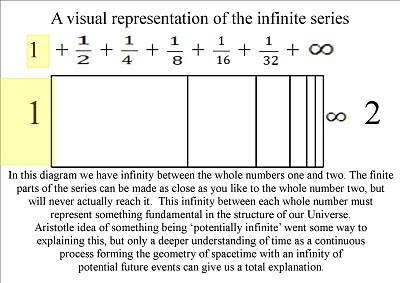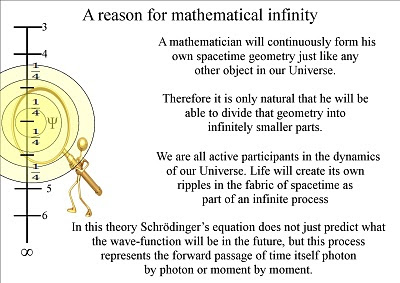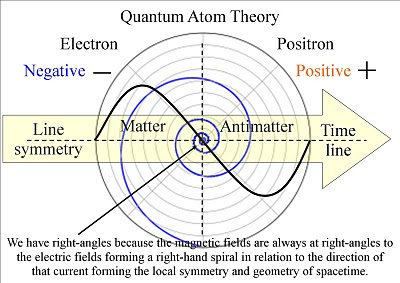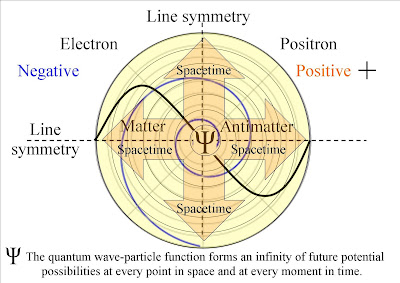Wednesday, 20 October 2010

Could the forward motion of light form the forward passage of time?

A link to the video 'Could our Universe be an infinity'

In this theory it is the inward absorption and outward emission of light that forms the arrow of time and geometry of spacetime. We live in a Universe of infinite possibilities because of the Probabilistic nature of light.In this theory each new moment in time is formed by a single photon electron coupling creating a wave-function of future possibilities.On the level of everyday objects the observer will see an infinite number of photon electron couplings, forming a temporary image of the Universe, photon by photon or moment by moment.We have an infinity of possibilities that will spiral out from every point in space and at every moment in time. This can explain the mathematical infinity that we have between each whole number.A mathematician will continuously form his own spacetime geometry by reacting with the wave particle duality of light. Therefore it is only logical that he will be able to divide that spacetime into infinitely smaller parts.This speculative diagram highlights the profound symmetry between matter and antimatter, positive and negative charge. We have wave-particle duality and the particle property of light and matter only manifests itself when something comes in contact with the wave.We have right-angles because the magnetic fields are always at right-angles to the electric fields forming a right-hand spiral in relation to the direction of the current forming the local symmetry and geometry of spacetime.In this theory our Universe is in continuous creation being formed by the inward absorption and outward emission of light or EMR. This forms matter antimatter annihilation forming positive and negative charge and electromagnetic fields.The quantum wave-particle function that is explained mathematically by Schrödinger’s wave equation forms an infinity of future potential possibilities at every point in space and at every moment in time, we see and feel this as the passage of time.We have a continuous process of symmetry forming and breaking that formed a mathematical base for the evolution of life. The continuous random breaking of this symmetry can be seen as Heisenberg’s Uncertainty Principle that is the same uncertainty that we have with any future event.

~